Science Journal of Applied Mathematics and Statistics
Volume 4, Issue 2, April 2016, Pages: 59-63

An Approach of Power Estimation for Linear Mixed Models for Clinical Studies

Weijia Feng

Alcon (China) Ophthalmic Product Co., Ltd., Beijing, China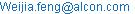Weijia Feng. An Approach of Power Estimation for Linear Mixed Models for Clinical Studies. Science Journal of Applied Mathematics and Statistics. Vol. 4, No. 2, 2016, pp. 59-63. doi: 10.11648/j.sjams.20160402.17

Received: February 25, 2016; Accepted: April 7, 2016; Published: April 11, 2016

Abstract: This study aims to demonstrate a practical way of power estimation for linear mixed models in clinical studies. Approximation methods using z and t statistics are discussed and compared to the simulated results. It was found that the approximation methods generally provide a slight overestimation of power, relative to simulated results using the Kenward and Roger estimation of degree of freedom. However, results of approximation methods can be informative in certain scenarios. In conclusion, the z approximation and t approximation with a residual degree of freedom can be useful in certain situations. Simulation method can serve as a general solution.

Keywords: Power, Sample Size, Linear Mixed Model, Clinical Studies

Contents

1. Introduction

Linear mixed models and generalized linear models are popular choices in the analyses of clinical trial data, especially for the studies with longitudinal designs (Cook and DeMets, 2007; Laird and Ware, 1982). At the study initiation stage, since the process of study planning is usually complex and involves various financial factors and parties, a quick estimation of sample size is considered very useful for the clinical team and decision makers.

Unfortunately, unlike the simpler cases of comparing group means or group proportions, such as classical t test of two sample means from normal populations, or the z test, for which the sample size calculation (Lachin, 1981; Chow et al., 2003; Julious, 2003) is even possible without using a calculator, the estimation of sample size for mixed linear models is typically complex and the approach is study-based (Dang et al., 2008; Chen et al., 2008; Vierron and Giraudeau, 2006; Heo et al., 2010; Kain et al., 2015; Candel and van Breukelen, 2015). Even for the cases of methods with some degree of generality (Lu et al., 2009; Liu and Liang, 1997; Self and Mauritsen, 1988), the actual implementation is more or less limited given their complexity.

In this report, the author presents an approach that is of a practical nature and show that approximation methods can be useful in the special, but definitely not rare cases, where the simplicity of estimation is important. Meanwhile as a general solution, a simulation method can be implemented which is not much more complicated than the proposed approximation methods.

2. Method

A general linear mixed model is defined as bellow: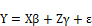(1)

Where Y is a n.. by 1 vector. n.. is the total number of observations. X is the design matrix for the fixed effects. The fix effects are represented by an m by 1 vector β, where m is the number of effects. Z is the coefficient for the random effect vector γ. Error term ε is a n. by 1 vector.

It is assumed that γ and ε are normally distributed with zero means. The covariance matrix between the random vectors is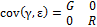, where G and R are the variance covariance matrix of γ and ε respectively. Zeros represent zero matrices of appropriate dimensions.

Define V as the variance-covariance matrix of y, then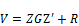(2)

In longitudinal clinical studies, usually researchers are primarily interested in the comparison of marginal means representing the treatment effect for all repeated measures (or visits), or for some repeated measures, or at one particular measure (Lu et al., 2009).Utilizing the mixed model, such marginal means can be compared by identifying and analyzing the linear combination of the fixed effect β, i.e.,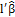, when a vector l is properly defined.

A typical two-sided test of equivalence can take the form H0: lβ=0 and H1: lβ≠0. Whereas a non-inferiority test could take the form H0: lβ≤Δ and H1: lβ>Δ. where Δ represents a non-inferiority margin.

To determine the power of such tests, a straightforward approach is to consider the following statistic: t =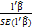(Lu et al., 2009; Heo et al., 2010), where SE standards for the standard error. When V (2) is unknown, the distribution of t is unknown (McCulloch and Searle, 2001). However, one can assume that using an estimate of V, t follows a central t distribution under the null hypothesis of the two sided test with an unknown degree of freedom (Rencher and Schaalje, 2008; Kenward and Roger, 1997). In such a manner, one can perform a t test with an estimated degree of freedom.

It is then natural to apply the method commonly used to determine the power of t tests to get the power estimates. This takes the approximate form (Chow et. al, 2008):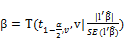(3)

where α, β are type I and type II error rates. T(X,v|B) is the cumulative t distribution function for X, with v degree of freedom and a non-centrality parameter of B.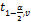is the (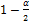)x 100-th quantile of the t distribution of v degree of freedom.

Alternatively, a Z test for large samples (Rencher and Schaalje, 2008) can be similarly defined by assuming an asymptotic normal distribution of the test statistic z =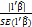, in this case the power can be approximately formulated as (Chow et. al, 2008),: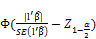(4)

where Φ is the cumulative distribution function of the standard normal variable.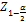is the ()x100-th quantile of the standard normal distribution.

Consider the maximum likelihood estimate of(Lu et al., 2009), the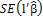can be specified (McCulloch and Searle, 2001) by taking the square root of(5)

The terms on the right hand side as well as the raw effect, are assumed to be known in the sense that the effect size is known, to be able to produce estimations of power.

3. A Simulation Study of a Typical Problem

In a hypothetical single-center, randomized study, subjects either receive the study drug or the placebo in a randomized manner, and are followed at two study visits. A continuous response (which could be the reduction of blood pressure, for example, if this is an antihypertensive drug study) is measured at the each of two study visits. A linear mixed model can be specified in the following manner using the (1):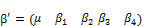,

where the last four elements of the vector represent the fixed effect for treatment of investigated drug and placebo, and the time effect of first visit and second visit, respectively. The model does not include an interaction effect for simplicity. Also this is for the consideration that an interaction effect is not always present for clinical trial data.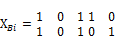If there is no missing observation, the submatrices of X can be arranged as above. The capitalized subscript indicates the treatment (A=Investigated drug, B=Placebo) and i means the i=th subject in the respective treatment group. If there are missing observations for a subject, the number of rows for submatrix corresponding to the subject will be less than the number of visits.

The matrix X can be defined as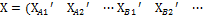). The Z matrix is of the block diagonal form: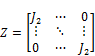,

where J2 is a 2 by 1 vector of 1s, if there is no missing observations. If there are missing observations, 1 should replace J2 if one of the two observations is missing.

A random effect of subject is defined. For simplicity, define that: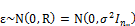where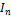is the identify matrix of the rank n, which is number of subjects.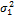and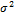are variance components.

Unless otherwise specified, simulations were performed by using the following parameters: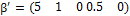,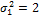,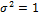.

In every scenario where the sample size, the treatment assignment ratio and the missing data proportion etc. are varied, 1000 samples were generated using the linear mixed model. Data were fitted using PROC MIXED in SAS 9.3 software with a correct specification of the model and various options of degree of freedom methods.

The least squares means (Khuri, 2010) of the effect "treatment" were estimated and a test of equivalence of the treatment least squares means was performed as described earlier in the text. The simulated power is the proportion of rejected tests to the total number of tests performed. Analytically, the difference of least squares means for the treatment effect is, where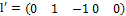.

4. Results

Table 1. Summary of estimated and simulated result of power for data without missing observations. N is the total number of subjects. Ratio is defined as number of subjects receiving the investigated drug divided by the number of subjects receiving placebo. LSDiff is the true difference of least squares means for the factor treatment, the other parameters are set as described in the main text. RES represents the residual degree of freedom. KD represents the Kenward Roger method for estimating the degree of freedom. Power means the estimated power for a given method. Simulation stands for the simulated power for a given method.

 N Df RES Df KD Median Power Z Power T RES Power T KD Simulation RES Simulation KD Ratio=1, LSDiff=1 20 37 18 0.293 0.280 0.267 0.29 0.266 50 97 48 0.609 0.60 0.591 0.621 0.603 80 157 78 0.807 0.803 0.798 0.804 0.801 100 197 98 0.885 0.882 0.872 0.871 0.869 Ratio=2, LSDiff=1 20 37 18 0.271 0.259 0.248 0.257 0.238 50 97 48 0.563 0.555 0.546 0.536 0.519 80 157 78 0.763 0.758 0.752 0.752 0.744 100 197 98 0.845 0.841 0.838 0.851 0.849 Ratio=1, LSDiff=0.5 20 37 18 0.105 0.102 0.099 0.138 0.124 50 97 48 0.20 0.197 0.194 0.208 0.197 80 157 78 0.293 0.29 0.287 0.27 0.267 100 197 98 0.352 0.35 0.347 0.312 0.305

Table 1 shows the power estimates using z test, and t test method for the data without missing observations. The degree of freedom based on the residual method is shown. The median of degree of freedom based on the Kenward-Roger (KR) method is also shown. The t estimation of power given KR degree of freedom is based on such a median. Taking the simulated power as the reference, the overestimation of power by z test method can be observed. For the estimated degree of freedom method, the residual method leads to an overestimation of power relative to the KR method. There is corresponding change of power when the parameters of the model changes. As an example, by comparing the block at the top with the block at the middle of table 1, the effect of ratio of subject number between two treatments on power can be identified. The block at the bottom of table 1 shows the drop of power when the effect size decreases.

Table 2. Summary of estimated and simulated results for power for data with missing observations. The missing mechanism is assumed to be missing completely at random. P missing stands for the probability of missing. N is the total number of subjects. Ratio is defined as number of subjects receiving the investigated drug divided by the number of subjects receiving placebo and is set to 1. LSDiff is the true difference of least squares means for the factor treatment, the other parameters are set as described in the main text. RES represents the residual degree of freedom. KD represents the Kenward Roger method for estimating the degree of freedom. Power means the estimated power for a given method. Simulation stands for the simulated power for a given method.

 N Df RES Df KD Median Power Z Power T RES Power T KD Simulation RES Simulation KD Ratio=1, LSDiff=1, P missing=0.1 20 37 18.1 0.293 0.280 0.267 0.29 0.266 50 97 47.98 0.609 0.60 0.591 0.621 0.603 80 157 78.0 0.807 0.803 0.798 0.804 0.801 100 197 97.9 0.885 0.882 0.872 0.871 0.869 Ratio=1, LSDiff=1, P missing=0.2 20 37 17.98 0.271 0.259 0.248 0.257 0.238 50 97 47.9 0.563 0.555 0.546 0.536 0.519 80 157 77.7 0.763 0.758 0.752 0.752 0.744 100 197 97.6 0.845 0.841 0.838 0.851 0.849

Table 2 demonstrates the power estimates when some of the observations are missing. In this case missing completed at random is assumed, i.e. the missing status of an observation is independent of the observed or non-observed value of the data. As the percentage of the missing data increases, there is a corresponding drop of power as predicted. In line with table 1, the overestimation of power by the z test method and the overestimation of the degree of freedom by residual degree of freedom are demonstrated. When the missing data is introduced, one of the results in simulation is that the estimated degree of freedom tends to deviate further from an integer value, as can be seen for the median degree of freedoms of the KR method.

5. Discussion

As demonstrated in the results, method of z test gives overestimation of the power. This is due to the assumption of large sample. Residual degree of freedom method is based on the assumption that correlational structure of the data is ignored (Schaalje et al., 2002). KR method is based on corrected estimation of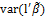, whereis the estimation of β, taking into account the underestimation by using the asymptotic variance covariance matrix () for, and the bias of estimation when the estimated variance covariance matrix is used in estimating(Kenward and Roger, 1997; McCulloch and Searle, 2001). There are other methods of identifying the degree of freedoms, such containment methods, Satterthwaite method (Schaalje et al., 2002), those methods are not evaluated in the current study.

Assume that the KR method is the method of choice in data analysis, it is obvious that the most general approach for the power estimation is to get simulated result using the very method. From the perspective of the t approximation method, simulation is basically needed to get the estimated degree of freedom. This is because that, for the Satterthwaite type of degree of freedom (Satterthwaite, 1946; Kenward and Roger, 1997; Rencher and Schaalje, 2008), both the estimated variance covariance matrix of y, and the sample variance covariance matrix of the variance components are needed besides the knowledge of other terms which are specified anyway for the effect size. Even if the estimated variance covariance matrix of y can be assumed known, the sample variance covariance matrix of the variance components would still need to be estimated typically by using the residual maximum likelihood (REML) method (Rencher and Schaalje, 2008).

In comparison, no simulation is needed for both the method of z approximation and residual degree of freedom. For the later, the only knowledge needed is the total number of observations and rank of X. In practice, those methods can become useful when the simulation is considered too time consuming. As has been demonstrated, even an overestimation of power is predictable, given the uncertainty already supplied by the effect size estimations, the overestimation may be regarded insignificant.

In terms of generality, as long as that the model parameters are fully defined, there is no restriction of the applicability besides those of the linear mixed models. The specification of design matrices will need some programming efforts for data with a desired pattern of imbalance, but those can usually be handled. The rest of operations, such as finding the rank of design matrices, and generalized inverses can all be automated as well using for example, PROC IML in SAS software. The l vector (as appeared in (2)) can be identified and checked for estimability. The G and R matrices can take any form, whenever they can be fully specified and well-defined.

6. Conclusion

The approximation of the power/ sample size for a linear mixed model can be flexible. When a quick estimation is needed with rough effect size estimates, the z approximation method and the t approximation with residual degree of freedom method can be used. The method of simulation with an appropriate choice of denominator degree of freedom can be used as a general solution.

References

1. N. Laird and J. Ware. Random-effects models for longitudinal data. Biometrics. 38(4), pp 963-974, Dec 1982.
2. A. Khuri. Linear Model Methodology. Taylor & Francis Group, 2010.
3. A. Rencher and G. Schaalje. Linear Models in Statistics, 2nd Edition. John Wiley & Sons, Inc., Hoboken, New Jersey. 2008.
4. C. McCulloch and S. Searle. Generalized, linear, and mixed models. John Wiley & Sons, Inc. 2001.
5. G. Schaalje, J. McBride and G. Fellingham. Adequacy of Approximations to Distributions of Test Statistics in Complex Mixed Linear Models Journal of Agricultural, Biological, and Environmental Statistics Vol. 7, No. 4, pp 512-524, 2002.
6. G. Liu and K. Liang. Sample Size Calculations for Studies with Correlated Observations. Biometrics Vol. 53, No. 3, pp 937-947, 1997.
7. M. Kenward and J. Roger. Small Sample Inference for Fixed Effects from Restricted Maximum Likelihood. Biometrics, Vol. 53, pp 983-997, 1997.
8. S. Julious. Sample sizes for clinical trials with normal data. Stat Med. 23(12), pp 1921-1986. 2004.
9. J. Lachin. Introduction to sample size determination and power analysis for clinical trials. Control Clin Trials. 2(2), pp 93-113, 1981.
10. E. Vierron and B. Giraudeau. Sample size calculation for multicenter randomized trial: taking the center effect into account. Contemp Clin Trials. 28(4), pp 451-458, 2007.
11. M. Heo, Y. Kim, X. Xue and M. Kim. Sample size requirement to detect an intervention effect at the end of follow-up in a longitudinal cluster randomized trial. Stat Med. 29(3), pp 382-390, Feb 2010.
12. M. Kain, B. Bolker and M. McCoy. A practical guide and power analysis for GLMMs: detecting among treatment variation in random effects. PeerJ. 3:e1226, Sep 2015.
13. M. Candel and G. van Breukelen. Sample size calculation for treatment effects in randomized trials with fixed cluster sizes and heterogeneous intraclass correlations and variances. Stat Methods Med Res. 24(5), pp 557-573, Oct 2015.
14. K. Lu, D. Mehrotra, G. Liu.Sample size determination for constrained longitudinal data analysis. Stat Med. 28(4), pp 679-699, 2009.
15. S. Self and R. Mauritsen. Power/Sample Size Calculations for Generalized Linear Models Biometrics Vol. 44, No. 1, pp 79-86, 1988.
16. Q. Dang, S. Mazumdar and P. Houckc. Sample Size and Power Calculations Based on Generalized Linear Mixed Models with Correlated Binary Outcomes. Comput Methods Programs Biomed. 91(2), pp 122–127, 2008.
17. J. Chen, S. Stock and C. Deng. Sample Size Estimation Through Simulation of a Random Coefficient Model by Using SAS. PharmaSUG. 2008.
18. T. Cook and D. DeMets. Introduction to Statistical Methods for Clinical Trials. Chapman and Hall/CRC, 2007.
19. S. Chow, J. Shao and H. Wang. Sample Size Calculations in Clinical Research. CRC, 2003.
20. F. Satterthwaite. An Approximate Distribution of Estimates of Variance Components. Biometrics Bulletin, Vol. 2, pp 110-114, 1946.

 Contents 1. 2. 3. 4. 5. 6.
Article ToolsAbstractPDF(203K)